# Cryptographic cipher-attack through Statistical Frequency Analysis

Alex Egg,

## Executive Summary

Throughout history, people have sought after ways to communicate in secret. This lead to the advent of ciphers to encode plaintext to ciphertext which can then, be reversed, and decoded to plaintext again. Some classical ciphers include: Caesar and Vigenere ciphers which provided secrecy for a while but eventually weaknesses were found and exploited. One weakness, which this paper will explore in detail, is exposed through the use of statistical frequency analysis. It turns out that, given a sample of english text, a constant letter frequency distribution forms. This is called the english fingerprint. It also turns out that this same fingerprint comes out in many ciphertexts allowing the ciphers to be decrypted without the correct key. In the search for perfect secrecy, this eventually lead to more and more sophisticated ciphers, such as modern day DES, AES and RSA. The use of statistics, particularly frequency analysis and distributions can be used to circumvent classical ciphers as well as modern day ciphers.

## Introduction

In cryptanalysis, frequency analysis is the study of the frequency of letters or groups of letters in a ciphertext. The method is used as an aid to breaking classical ciphers.

Frequency analysis is based on the fact that, in any given stretch of written language, certain letters and combinations of letters occur with varying frequencies. Moreover, there is a characteristic distribution of letters that is roughly the same for almost all samples of that language. For instance, given a section of English language, E, T, A and O are the most common, while Z, Q and X are rare. Likewise, TH, ER, ON, and AN are the most common pairs of letters, and SS, EE, TT, and FF are the most common repeats. The nonsense phrase “ETAOIN SHRDLU” represents the 12 most frequent letters in typical English language text.

In some ciphers, such properties of the natural language plaintext are preserved in the ciphertext, and these patterns have the potential to be exploited. 

This paper hopes to demonstrate that though statistical frequency analysis that is possible to perform cipher attacks on classic encryption ciphers (such as substitution), and even possibly modern ciphers like DES.

This paper employs the use of many tools to explore the vulnerabilities of the various ciphers introduced:

• Matlab scripts are used to generate frequency distributions (histograms)
• Cipher implementations are written in the Ruby language
• Various techniques of probability and combinatorics are used to analyze the ciphers

The project is intended to take approximately 2 weeks of work. I will first have to research and learn about cryptography basics, especially the classic ciphers and if time/complexity permits I will explore modern and more complicated ciphers and their weaknesses. I must also allocate time to implement the various ciphers in Ruby language to run analysis and experiments. Time also must be taken to write matlab scripts and brush up on probability theory.

## Background Research/History

The first well known cipher, a substitution cipher, was used by Julius Caesar around 58 BC. It is now referred to as the Caesar cipher. Caesar shifted each letter in his military commands in order to made them appear meaningless should the enemy intercept it.

Imagine Alice and Bob decide to communicate using the Caesar cipher. First, they would need to agree in advance on a shift to use, say 3, so to encrypt her message Alice will need to apply a shift of 3 to each letter in her original message, so A becomes D, B becomes E, C becomes F, and so on. This unreadable or encrypted message, is then sent to Bob openly. Then Bob simply subtracts the shift of 3 from each letter in order to read the original message. 

Incredibly, this basic cipher was used by military leaders for hundreds of years after Caesar. However, a lock is only as strong as its weakest point, a lockbreaker may look for mechanical flaws or failing that extract information in order to narrow down the correct combination. The process of lock breaking and code breaking are very similar. The weakness of the Caesar cipher was published 800 years later by an Arab mathematician named Al-Kindi. He broke the Caesar cipher by using a clue based on an important property of the language the message was written in. If you scan text from any book and count the frequency of each letter, you’ll find a fairly consistent pattern. For example, these are the letter frequencies of English: “ (Khan Academy)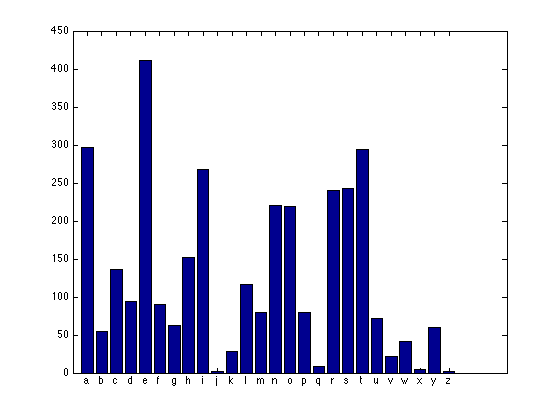Figure 2: Letter frequency distribution of the text in this paper. See Appendix A for the matlab script to generate this histogram.

This can be thought of as a fingerprint of English. We leave this fingerprint when we communicate without realizing it. This clue is one of the most valuable tools for a codebreaker. To break this cipher, they count up the frequencies of each letter in the encrypted text and check how far the fingerprint had shifted. For example, if H is the most popular letter in the encrypted message instead of E, then the shift was likely 3. So they reverse the shift in order to reveal the original message. This is called frequency analysis and that was a blow to the security of the Caesar cipher. 

## Substitution Ciphers (Caesar Cipher)

Technically, the Caesar cipher is a circular permutation which, given an arbitrary shift K, replaces each letter of the plaintext by the letter K places later.

### Frequency Analysis

Suppose Bob wants to send Alice an encrypted message. He chooses a simple substitution (Caesar) cipher: shift every character 3 letters to the right. His plaintext message:

Sandman is in America keep Brody in play and contact Kerry. This is an urgent matter so delete this message


shift 3 cipher text message:

Vdqgpdq lv lq Dphulfd nhhs Eurgab lq sodab dqg frqwdfw Nhuuab1 Wklv lv dq xujhqw pdwwhu vr ghohwh wklv phvvdjh


Eve is a spy and intercepts Bob’s ciphertext message sent to Alice. She uses frequency analysis break the cipher:

Observe the character frequency distribution of the cipher text transposed over the baseline distribution presented above: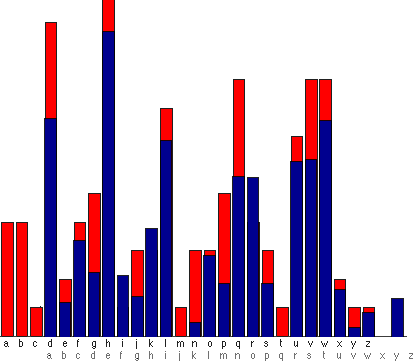Figure 3: The original “fingerprint” distribution is superimposed on top of the shifted distribution to highlight the fact that the same distribution is present in the ciphertext, but shifted 3 letters.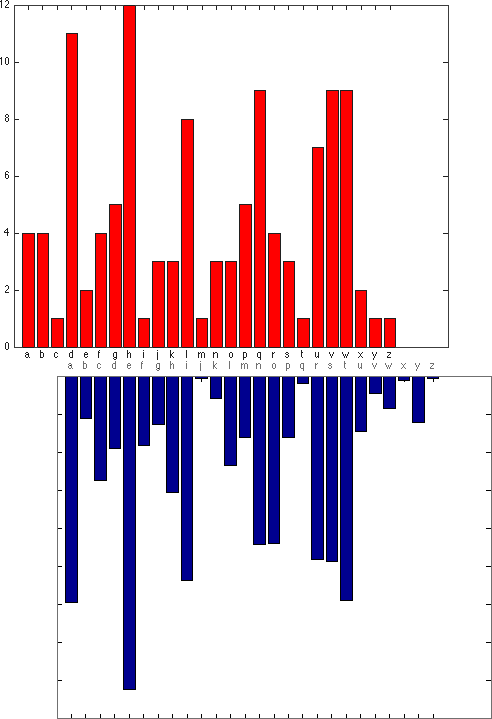Figure 4: The same dataset as the previous figure but displayed differently to show how the original distribution (blue) comes out in the ciphertext distribution only shifted over to the left 3 spots.

It is apparent that if you shift the character distribution from the ciphertext (red) to the left 3 character positions, it closely matches the fingerprint for the English language, i.e., ciphertext ‘h’ is plain text ‘e’ and so on, thusly bob shifted his message 3 characters to the right. Eve can now decrypt Bob’s ciphertext. This is called a ciphertext attack.

### Brute Force Analysis

Another interesting point about the Caesar Cipher is that for any given plain text, there can only be 26 possible ciphers generated. This is because it can either shifted once, twice… or 26 times. Thus, given the above example ciphertext:

Vdqgpdq lv lq Dphulfd nhhs Eurgab lq sodab dqg frqwdfw Nhuuab1 Wklv lv dq xujhqw pdwwhu vr ghohwh wklv phvvdjh


It would be a trivial task with today’s computer power to brute force all the possible combinations of shifts (26) to get the plain text. Below is an example output of all possible combinations:

Running “sub.rb”…
ruby 1.9.2p290 (2011-07-09 revision 32553) [x86_64-darwin11.0.1]
"Sandman is in America keep Brody in play and contact Kerry. This is an urgent matter so delete this message"
"shift=0: Vdqgpdq lv lq Dphulfd nhhs Eurgb lq sodb dqg frqwdfw Nhuub1 Wklv lv dq xujhqw pdwwhu vr ghohwh wklv phvvdjh"
"shift=1: Ucpfocp ku kp Cogtkec mggr Dtqfa kp rnca cpf eqpvcev Mgtta0 Vjku ku cp wtigpv ocvvgt uq fgngvg vjku oguucig"
"shift=2: Tboenbo jt jo Bnfsjdb lffq Cspe jo qmb boe dpoubdu Lfss/ Uijt jt bo vshfou nbuufs tp efmfuf uijt nfttbhf"
"shift=3: Sandman is in America keep Brod_ in play and contact Kerry. This is an urgent matter so delete this message"
"shift=4: Rmclm hr hm @ldqhb jddo Aqnc^ hm ok^ mc bnmsbs Jdqq^- Sghr hr m tqfdms lssdq rn cdkdsd sghr ldrrfd"
"shift=5: Q_lbk_l gq gl ?kcpga_ iccn @pmb] gl nj_] _lb amlr_ar Icpp], Rfgq gq _l speclr k_rrcp qm bcjcrc rfgq kcqq_ec"
"shift=6: P^kaj^k fp fk >jbof^ hbbm ?ola\\ fk mi^\\ ^ka lkq^q Hboo\\+ Qefp fp ^k rodbkq j^qqbo pl abibqb qefp jbpp^db"
"shift=7: O]ji]j eo ej =iane_] gaal >nk[ ej lh][ ]j _kjp]_p Gann[* Pdeo eo ]j qncajp i]ppan ok ahapa pdeo iaoo]ca"
"shift=8: N\\i_h\\i dn di <hmd^\\ fk =mj_Z di kg\\Z \\i_ ^jio\\^o FmmZ) Ocdn dn \\i pmbio h\\oom nj _go ocdn hnn\\b"
"shift=9: M[h^g[h cm ch ;g_lc][ e__j <li^Y ch jf[Y [h^ ]ihn[]n E_llY( Nbcm cm [h ola_hn g[nn_l mi ^_f_n_ nbcm g_mm[a_"
"shift=10: LZg]fZg bl bg :f^kb\\Z d^^i ;kh]X bg ieZX Zg] \\hgmZ\\m D^kkX' Mabl bl Zg nk^gm fZmm^k lh ]^e^m^ mabl f^llZ^"
"shift=11: KYf\\eYf ak af 9e]ja[Y c]]h :jg\\W af hdYW Yf\\ [gflY[l C]jjW& Lak ak Yf mj_]fl eYll]j kg \\]d]l] lak e]kkY_]"
"shift=12: JXe[dXe j e 8d\\iZX b\\\\g 9if[V e gcXV Xe[ ZfekXZk B\\iiV% K_j j Xe li^\\ek dXkk\\i jf [\\c\\k\\ k_j d\\jjX^\\"
"shift=13: IWdZcWd _i _d 7c[h_YW a[[f 8heZU _d fbWU WdZ YedjWYj A[hhU\$ J^_i _i Wd kh][dj cWjj[h ie Z[b[j[ j^_i c[iiW]["
"shift=14: HVcYbVc ^h ^c 6bZg^XV ZZe 7gdYT ^c eaVT VcY XdciVXi @ZggT# I]^h ^h Vc jg\\Zci bViiZg hd YZaZiZ i]^h bZhhV\\Z"
"shift=15: GUbXaUb ]g ]b 5aYf]WU _YYd 6fcXS ]b dUS UbX WcbhUWh ?YffS\" H\\]g ]g Ub if[Ybh aUhhYf gc XYYhY h\\]g aYggU[Y"
"shift=16: FTaWTa \\f \\a 4Xe\\VT ^XXc 5ebWR \\a c_TR TaW VbagTVg >XeeR! G[\\f \\f Ta heZXag TggXe fb WX_XgX g[\\f XffTZX"
"shift=17: ESV_S [e [ 3_Wd[US ]WWb 4daVQ [ b^SQ SV UafSUf =WddQ  FZ[e [e S gdYWf _SffWd ea VW^WfW fZ[e _WeeSYW"
"shift=18: DR_U^R_ Zd Z_ 2^VcZTR \\VVa 3cUP Z_ a]RP R_U T_eRTe <VccP\u001F EYZd Zd R_ fcXV_e ^ReeVc d UV]VeV eYZd ^VddRXV"
"shift=19: CQ^T]Q^ Yc Y^ 1]UbYSQ [UU 2b_TO Y^ \\QO Q^T S_^dQSd ;UbbO\u001E DXYc Yc Q^ ebWU^d ]QddUb c_ TU\\UdU dXYc ]UccQWU"
"shift=20: BP]S\\P] Xb X] 0\\TaXRP ZTT_ 1a^SN X] _[PN P]S R^]cPRc :TaaN\u001D CWXb Xb P] daVT]c \\PccTa b^ ST[TcT cWXb \\TbbPVT"
"shift=21: AO\\R[O\\ Wa W\\ /[SWQO YSS^ 0]RM W\\ ^ZOM O\\R Q]\\bOQb 9SM\u001C BVWa Wa O\\ cUS\\b [ObbS a] RSZSbS bVWa [SaaOUS"
"shift=22: @N[QZN[ V V[ .ZR_VPN XRR] /_\\QL V[ ]YNL N[Q P\\[aNPa 8R__L\e AUV V N[ b_TR[a ZNaaR_ \\ QRYRaR aUV ZRNTR"
"shift=23: ?MZPYMZ U_ UZ -YQ^UOM WQQ\\ .^[PK UZ \\XMK MZP O[ZMO 7Q^^K\u001A @TU_ U_ MZ a^SQZ YMQ^ _[ PQXQQ TU_ YQ__MSQ"
"shift=24: >LYOXLY T^ TY ,XP]TNL VPP[ -]ZOJ TY [WLJ LYO NZY_LN_ 6P]]J\u0019 ?ST^ T^ LY ]RPY_ XL__P] ^Z OPWP_P _ST^ XP^^LRP"
"shift=25: =KXNWKX S] SX +WO\\SMK UOOZ ,\\YNI SX ZVKI KXN MYX^KM^ 5O\\\\I\u0018 >RS] S] KX _\\QOX^ WK^^O\\ ]Y NOVO^O ^RS] WO]]KQO"
"shift=26: <JWMVJW R\\ RW *VN[RLJ TNNY +[XMH RW YUJH JWM LXW]JL] 4N[[H\u0017 =QR\\ R\\ JW ^[PNW] VJ]]N[ \\X MNUN]N ]QR\\ VN\\\\JPN"
Program exited with code #0 after 0.06 seconds.


Figure 5: Output from ruby script that brute forces all the possible shift combinations. Shift 0 is the original ciphertext. You’ll notice on shift 3 the plaintext message is exposed!

## Polyalphabetic Ciphers (Vigenere)

A strong cipher is one which disguises your fingerprint. To make a lighter fingerprint is to flatten this distribution of letter frequencies.
By the mid-15th century, we had advanced the polyalphabetic ciphers to accomplish this. Imagine Alice and Bob shared a secret shift word. First, Alice converts the word into numbers according of the letter position in the alphabet. Next, this sequence of numbers is repeated along the message. Then each letter in the message is encrypted by shifting according to the number below it. Now, she’s using multiple shifts instead of a single shift across the message as Caesar had done before.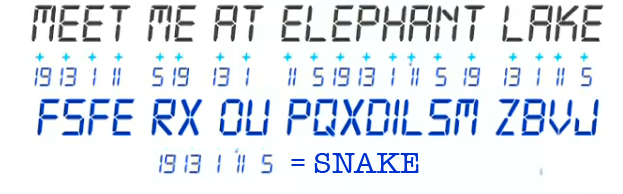Figure 6: The key ‘snake’ encodes to [19, 13, 1, 11, 5], now these are your shifts. Instead of just one shift with Caesar. Now just continually iterate through your shifts and encode the plaintext. This essentially makes a one cipher out of 5 Caesar Ciphers.

Then, the encrypted message is sent openly to Bob. Bob decrypts the message by subtracting the shifts according to the secret word he also has a copy of.
Bob meets w/ Alice a few days in advance and leaves her the secret shift word “snake”. Then a few days later Bob sends the below ciphertext to Alice:

Meet me at elephant lake, make sure nobody follows you and bring the bomb

fsferxoupqxdilsmzbvjfolpxnffytucejkhzmzblmpfgkworyasczru


### Frequency Analysis

Eve intercepts the ciphertext again, can she still use frequency analysis to decrypt it? When Eve calculates the letter frequencies, she’ll find a flatter distribution or a lighter fingerprint, so how can she break this?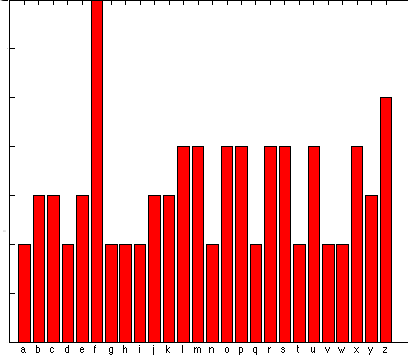Figure 7: Compare this distribution to that generated from the Caesar Cipher. This one is remarkably more uniform (flat). The uniformity is a result from using not 1 shift but 5.

We recall that a Caesar K-shift is the circular permutation which replaces each letter of the alphabet by the letter K places later (with wrap around). In Vigenere encryption, the key consists of a period P and a sequence K1,K2,…,KP of Caesar shifts. This given, the plaintext is broken up into successive strings of P letters each and the sth letter of each string is replaced by its image under the Caesar Ks-shift.
This encryption system is vulnerable to letter frequency analysis. The letter frequencies observed in the sequence of sth letters have the same distribution as the plaintext letters only Ks-shifted.
To break Vigenere encryption, guesses a period P and then, by comparing the histogram of observed frequencies of sth letters to the histogram of English letter probabilities, one is led to the correct value of Ks. A wrong guess for the period P leads to relatively flat histograms for all or most of the values of s. The code breaker in this case repeats the analysis with a new trial period. 

Even though the distribution is seemingly flat his distribution still leaks information. Any time there’s a differential in letter frequencies, a leak of information occurs. This difference is caused by repetition in the encrypted message. In this case, Alice’s cipher contains a repeating code word. In the above example, when she checks the frequency distribution of every fifth letter, the fingerprint will reveal itself. Consider only a portion of the ciphertext “Meet me at elephant lake”: Through experimentation Eve has determined that it is a 5-character secret key so she takes samples of every fifth character: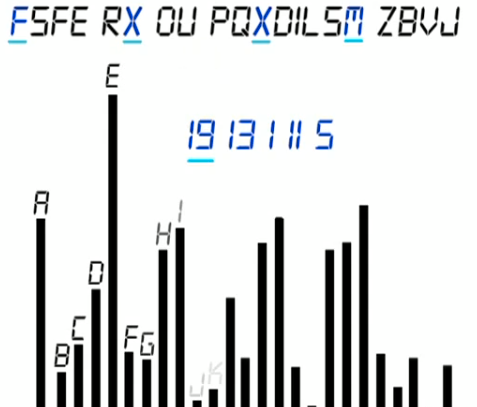Figure 8: Observe that the combination of every fifth character in the above distribution creates 1 caesar cipher.

Now, the problem and difference is to break five Caesar ciphers in a repeating sequence. Individually, this is a trivial task as we have seen before, the added strength of the cipher is the time taken to determine the length of shift word used. The longer the shift word, the stronger the cipher.
The following figuire is an example of the individual distributions for each shift from the samples above. It is like we have 5 individual Ceasar ciphers making up 1 cipher: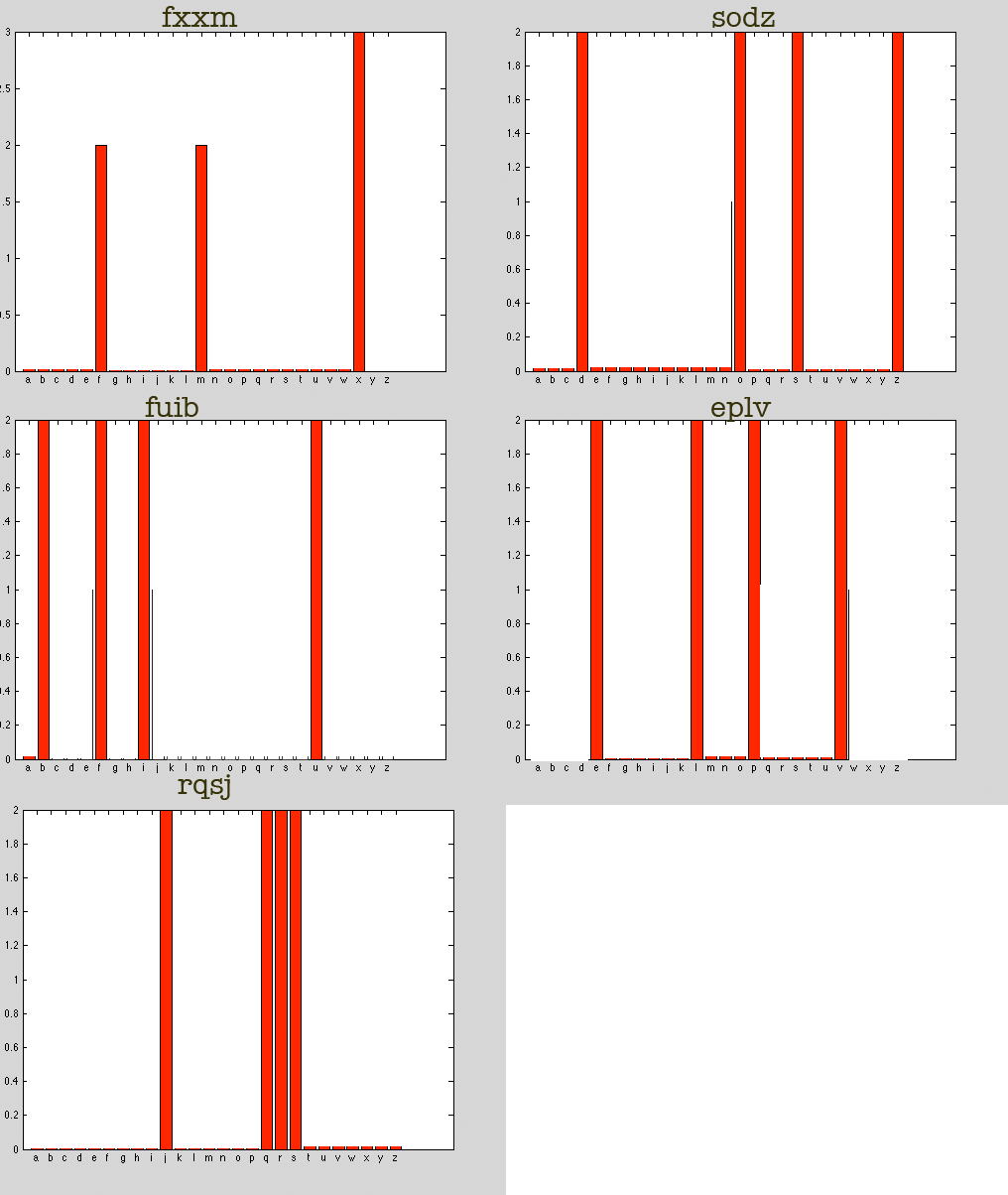Figure 9: These are the 5 Caesar cipher subsamples that make up the Vigenere Cipher above.

As you can see it is hard to find the english language fingerprint in these small sample distributions. If we took the whole ciphertext you would have 11 samples in each distribution above making it easier to find the shift. In general it is difficult to find the shift if the ciphertext is shorter than 50 characters; and as a rule of thumb: a longer plaintext means a longer the ciphertext which results in larger sample for a codebreaker to work with. Thusly, it is always in Bob and Alice’s best interest to compress messages before encryption. For example, single letter messages are very hard to break!

### Brute Force Analysis

The polyalphabetic cipher is a modification of the ceasar cipher. In the ceasar cipher every character is shifted by a constant x, resulting in only 26 possible combinations. With a polyalphabetic cipher, however, the shift is different for each character. Thusly for each character in a x character message there are possible shifts – exponential growth. For example given a 10 character plaintext the total possible ciphers to guess would be 10^26=1.41167095653376 × 10^14 which is highly improbable.

For over four hundred years, the problem remained. How could Alice design a cipher that hides her fingerprint, thus, stopping the leak of information. The answer is randomness. Imagine Alice rolled a 26 sided-dice to generate a long list of random shifts, and share this with Bob, instead of a code word (like snake). Now, to encrypt her message, Alice uses the list of random shifts instead. It’s important that this list of shift is long as the message as to avoid any repetition. Then, she sends it to Bob, who decrypts the message using the same list of random shifts she’d given him. Now Eve will have a problem, because the resulting encrypted message will have two powerful properties:
the shifts never fall into a repetitive pattern (like ‘snake’)
the encrypted message will have a uniform frequency distribution
because there’s no frequency differential, and therefore no leak, it’s now impossible for Eve to break the encryption. This is the strongest possible method of encryption, and it emerges toward the end of the 19th century, it’s now known as the one time pad.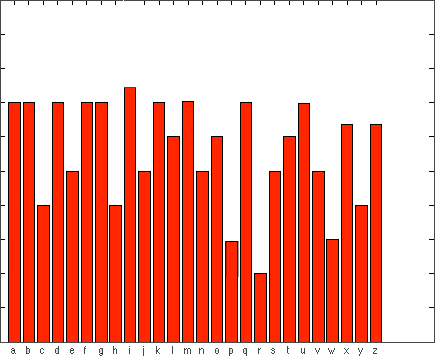Figure 10: The frequency distribution for the one-time pad. As the ciphertext becomes longer the distribution becomes more and more uniform (flat).

In order to visualize the strength of the one time pad, we must understand the combinatorial explosion which takes place. For example, the Caesar cipher shifted every letter by the same shift, which was some number between 1 and 26. So if Alice was to encrypt her name, it would result in 1 of 26 possible encryptions, a small number of possibilities, easy to check them all, known as brute force search. Compare this to the one time pad, where each letter would be shifted by a different number between 1 and 26, Now think about the number of possible encryptions, it’s going to be 26 multiplied by itself 5 times, which is almost 12 millions. Sometimes it’s hard to visualize. 
So imagine she wrote her name on a single page, and on top of it, stack every possible encryption. How high do you think this would be? With almost 12 million possible five letter sequences, this stack of paper would be enormous, over one kilometer high. When Alice encrypts her name using the one time pad, it’s the same as picking one of these pages at random, from the perspective of Eve, the codebreaker, every five letter encrypted word she has is equally likely to be any word in this stack. So, this is perfect secrecy in action. 
The WWII German Enigma Machine is an implementation of one-time pad, but with a key period of about 16,900.
This type of encryption is called asymmetric-key encryption and will lead us into the world of modern cryptography.

## Modern Ciphers

The work for perfect secrecy and minimal information leak continued into the 20th century with the advent of one of the first modern ciphers: DES. Designed at IBM in 1975 and based on Lucifer; it was designed by Feistel and Coppersmith. It is a hybrid of the substitution (Caesar) and permutation (one-time pad) ciphers.
It turns out that frequency analysis of most modern ciphers is a difficult target to hit as they leak little or no information. Most seem to be a hybrid of substitution and polyalphabetic cause extremely flat frequency distributions. I more thorough study of the modern ciphers would be required to explore their weaknesses and if frequency analysis is even an option. However, a full look into modern ciphers is beyond the scope of this paper; however, topics to research individually are:

• DES
• AES
• Diffie-Hellman
• RSA
• Block Ciphers

## Frequency Stability/Randomness

Most computer generated randoms are pseudorandom. In order for one-time pad or other asymmetric-key ciphers to be secure, the secret keys must truly be random. Pseudo random keys/shifts leak information.
This pseudorandom leaking phenomenon was explored during the first week of class with the coin toss experiment. Some students flipped a coin 100 times while others tried to fake the results of 100 flips. It became apparent that true randomness is really only possible with nature – the vast majority of students could not produce that flat frequency distribution that true coin tosses produce. This is an example of a uniform binomial distribution.
The above experiment can also be described by the following experiment from Khan Academy. Consider the following: imagine two rooms, inside each room is a switch. In one room, there’s a man who flips his switch according to a coin flip. If he lands head, the switch is on and if he lands tail, the switch is off. In the other room, a woman switches her light based on a blind guess. She tries to simulate randomness without a coin. Then we started a clock, and they made their switches in unison. Can you determine which light bulb is being switched by a coin flip? The answer is Yes. But how? And the trick is to think a property of each sequence rather than looking for any specific patterns. For example, first we may try to count the number of 1s and 0s, which occur in each sequence. This is close, but not enough, since they’re both seem fairly even. The answer is to count sequences of numbers, such as runs of three consecutive switches. A true random sequence will be equally likely to contain every sequence of any length. This is called a frequency stability property and is demonstrated by the uniform graph demonstrated by the one-time pad cipher text. The forgery is now obvious. Humans favored certain sequences when they make guesses resulting in uneven patterns, such as we see here. One reason that this happens, because we make the mistakes of thinking certain outcomes are less random than others. But realize there’s no such thing as a lucky number. There’s no such thing as a lucky sequences. If we flip a coin 10 times, is equally likely to come up all heads, all tails, or any other sequences you can think of. 
Once again, this leads us to an important concept of encryption: true randomness. If we look at the frequency distribution of a one-time pad key, if the distribution is not uniform, then it is not truly random and the resulting ciphertext will leak information.

## Conclusion

The results of our tests conclude that a number of the classical ciphers are vulnerable to frequency analysis attacks because they leak information. Especially longer ciphertext – the longer the length of the ciphertext the easier the attack. However, more advanced ciphers like the one-time pad are more resilient to frequency analysis because of an essentially flat uniform frequency distribution. And modern ciphers like DES or AES are impervious to frequency analysis because they are a hybrid of the classical ciphers and new techniques that do not leak information. More recently the advent of “s-boxes” – which remove linearity form ciphers – has proved frequency analysis even more futile on modern ciphers. Additionally, a hallmark of modern ciphers is the very large key space which render brute force techniques ineffective.
In summary, the applications of statistical analysis are profound and surround us everywhere in practical application in day-to-day life. I have a new appreciation for the power of analysis and this project was a valuable learning experience. I feel I know have a better understanding of the statistics/probability presented in this course and a better understanding of basic cryptography. In the future if I were to conduct this experiment again I would allot time to focus more on modern ciphers that are actually in use today – that is the only thing I would do differently. I also plan on taking a computer security class next semester in the CS department to explore modern ciphers in more detail.

### Additional Applications of Frequency Analysis

While I was writing the matlab script to do the frequency histograms, I had the epiphany, “this is how Google translate can detect what language I’m typing”. The Google translate page allows you to type one language and then it’ll translate it to another. It doesn’t need to know what the input language is, it detects it automatically. I’ve always wondered how they do this. It’s intuitive how it can tell English from Korean – it’s a completely different character set. However, how can it tell English from Spanish? My conclusion is that it does some type of frequency analysis of the input and compares it to reference distributions it has in a database.
Similarly, the Mac OS X Core Foundation Framework APIs also have a function that tries to guess the language of a string. I ran into this API years ago and have always remembered it because it seemed like magic:

### CFStringTokenizerCopyBestStringLanguage

Guesses a language of a given string and returns the guess as a BCP 47 string.

CFStringRef CFStringTokenizerCopyBestStringLanguage (

CFStringRef string,

CFRange range
);

##### Parameters

string

The string to test to identify the language.

range

The range of string to use for the test. If NULL, the first few hundred characters of the string are examined.

##### Return Value

A language in BCP 47 form, or NULL if the language in string could not be identified. Ownership follows the Create Rule in Memory Management Programming Guide for Core Foundation.

##### Discussion

The result is not guaranteed to be accurate. Typically, the function requires 200-400 characters to reliably guess the language of a string.

Figure 11: Excerpt from OSX API documentation about an internal API that does language detection

From the documentation is specifies “the function requires 200-400 characters to reliably guess the language of a string”. From my research above, I can hypothesis the reason for this requirement is so the algorithm can generate an accurate frequency distribution to make the language inference. Although, from my experiments it typically only requires >50 characters.
I have gained a more solid understanding of statistics and probability in writing this paper, but they still seem like magic to me. That is a good thing.

 https://developer.apple.com/library/mac/#documentation/CoreFoundation/Reference/CFStringTokenizerRef/Reference/reference.html
 http://en.wikipedia.org/wiki/Frequency_analysis
 http://en.wikibooks.org/wiki/Cryptography/Frequency_analysis
 http://www.mathworks.com/matlabcentral/fileexchange/31522-substitution-cipher-encoder-and-decoder/content/subdecode.m
 http://math.ucsd.edu/~crypto/java/EARLYCIPHERS/Vigenere.html

## Appendix

### Code Listings

sub.rb – Caesar Cipher implementation in Ruby

class SubCrypt
def decrypt(c, shift)
crypt(c, -shift)
end
def crypt(m, shift)
c=""
m.each_char do |char|
(c << " " && next) if char == " "
shifted_char = char.ord+shift
shifted_char=(shifted_char % 122)+96 if (shifted_char > 122)
c << shifted_char
end
return c
end
end
shift=+3
p m="Sandman is in America keep Brody in play and contact Kerry. This is an urgent matter so delete this message"
c="#{SubCrypt.new.crypt(m, shift)}"
p "shift=0: #{c}"
(1..26).each do |offset|
p "shift=#{offset}: #{SubCrypt.new.decrypt(c, offset)}"
end
# "Vdqgpdq lv lq Dphulfd nhhs Eurgab lq sodab dqg frqwdfw Nhuuab1 Wklv lv dq xujhqw pdwwhu vr ghohwh wklv phvvdjh"


poly.rb – Vegenre Cipher implementation in Ruby

#this is the polyalphabtic cipher implementation
#to make this one-time pad just make the shared_secret random and the length of the message
m="Meet me at elephant lake Make sure nobody follows you Bring the bomb this thing is acting rally stupid it should b a compltly flat distribution".downcase!.delete!(" ")
# shared_secret="snake"
shared_secret = "pbzakqmustwtbdzevbydfuvbyvihwasghqiqzrvqbfwgwjmturdrduzcbbeufjaleivuznxuiqdndndpjuiqnvnlzsxxagcsqqpbrdnogsnnbssapxwfyptcxjxlhvbabxsmjopcnxganoe"# (0...m.length).map{96.+(rand(26)).chr}.join
p shared_secret
cipher=""
m.chars.each_with_index do |c, i|
shift = shared_secret[i % shared_secret.length].ord-96
shifted_char = c.ord+shift
shifted_char=(shifted_char % 122)+96 if (shifted_char > 122)
cipher << shifted_char.chr
end
p m
p cipher


freq_dist.m – Matlab script to generate frequency distributions

 fileTarget = 'sample_english.txt';
x = char(x);
x = lower(x);
% x = 'abcdefghijklmnopqrstuvwxyz';
% x=lower(x);
x = sort(x);
[U I J] = unique(x);

N = diff([0 I]);
x
bar(N, 'red')
U
set(gca,'XTick',[1:26])
set(gca,'XtickLabel',U.')